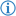The subtract operator is an arithmetic operator which subtracts a number object from another.

Its token is named SUBTRACTION.

## Phrases

• `minus`
• `without`
• `the difference between`
• `and`
• `subtract`
• `from`

### InfixInfix notation can be achieved using the phrases `minus` or `without`.

Its FDS is

#### Example

`I wrote`` ``the number`` ``13`` ``minus`` ``the number`` ``10``.`

This prints `3`.

Its Java equivalent is as follows:

```System.out.println((double)13 - (double)10);
```

### PrefixPrefix notation can be achieved using the phrase `subtract` or `the difference between` and the infix separator `from` or `and`.

Its FDS is {{codeblock|`[subtract/the difference between]``<whitespace>``<value:number>``<whitespace>``[and/from]``<whitespace>``<value:number>`

#### Example

`I wrote`` ``the difference between`` ``the number`` ``13`` ``and`` ``the number`` ``10``.`

This has the same effect as the previous example.

Note that this can introduce some ambiguity. For instance:

`I wrote`` ``the difference between`` ``10``and`` ``2`` ``and``5``.`
13

One might expect the output to be 3, because they expect its Java equivalent to be

```10 - 2 - 5
```

, but its Java equivalent is actually

```10 - 2 + 5
```

. This is because the `the difference between` and `subtract` operators only have one partner infix operator, `and` and `from`, respectively. This means that

{{codeblock|`I wrote`` ``the difference between`` ``10``and`` ``2` is processed alone, and returns `8`. Then, `8`` ``and`` ``5` is processed as addition, returning `13`. This is because using an `and` as an operator without any prefix context denotes addition. There is no prefix context for the second `and` because the first one has already been processed.

## DecrementMane article: Decrement

The decrement operator is a special case of subtraction, and subtracts the fixed value `1` from a variable and cannot be used on literals.